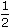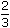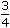# Aptitude - Ratio and Proportion

Exercise : Ratio and Proportion - General Questions
6.
The ratio of the number of boys and girls in a college is 7 : 8. If the percentage increase in the number of boys and girls be 20% and 10% respectively, what will be the new ratio?
8 : 9
17 : 18
21 : 22
Cannot be determined
Explanation:

Originally, let the number of boys and girls in the college be 7x and 8x respectively.

Their increased number is (120% of 7x) and (110% of 8x).120 x 7xand110 x 8x100 10042x and 44x 5 5The required ratio =42x : 44x= 21 : 22. 5 5

7.
Salaries of Ravi and Sumit are in the ratio 2 : 3. If the salary of each is increased by Rs. 4000, the new ratio becomes 40 : 57. What is Sumit's salary?
Rs. 17,000
Rs. 20,000
Rs. 25,500
Rs. 38,000
Explanation:

Let the original salaries of Ravi and Sumit be Rs. 2x and Rs. 3x respectively.

 Then, 2x + 4000 = 40 3x + 4000 5757(2x + 4000) = 40(3x + 4000)6x = 68,0003x = 34,000

Sumit's present salary = (3x + 4000) = Rs.(34000 + 4000) = Rs. 38,000.

8.
If 0.75 : x :: 5 : 8, then x is equal to:
1.12
1.2
1.25
1.30
Explanation:

 (x x 5) = (0.75 x 8)x =6= 1.20 5

9.
The sum of three numbers is 98. If the ratio of the first to second is 2 :3 and that of the second to the third is 5 : 8, then the second number is:
20
30
48
58
Explanation:

Let the three parts be A, B, C. Then,

 A : B = 2 : 3 and B : C = 5 : 8 =5 x 3:8 x 3= 3 : 24 5 5 5A : B : C = 2 : 3 : 24 = 10 : 15 : 24 5B =98 x 15= 30. 49

10.
If Rs. 782 be divided into three parts, proportional to::, then the first part is:
Rs. 182
Rs. 190
Rs. 196
Rs. 204
Given ratio =::= 6 : 8 : 9.1st part = Rs.782 x 6= Rs. 204 23